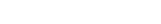# Overview (in Hindi)

The course deals with equations with one unknown variable. Linear equations are equations of the first degree, meaning that the unknown variable appears with an exponent of 1. They can always be brought to the form ax + b = 0 where a, b are constants and a ≠ 0. They have one root (solution) which is x = -b/a. The graph of their underlying function f(x) = ax + b is a straight line, hence their name. Quadratic equations are equations of the second degree, meaning that the unknown variable appears with an exponent of 2. They can always be brought to the form ax² + bx + c = 0 where a, b, c are constants and a ≠ 0. The constants a, band c form a quantity D = b² - 4ac, aptly called the Determinant, which determines whether the equation has any real roots. (This results from the fact that the solution contains the square root of D, which isn’t a ‘real’ number for D < 0.) If D >= 0, quadratic equations have two real roots (merged into one for D = 0), which can be calculated by the formula: x₁‚ ₂ = (-b ±√D)/2a The graph of their underlying function f(x) = ax² + bx + c is a parabola whose arms tend to f(x) = +∞ for a > 0 and to f(x) = -∞ for a < 0. They take their name (quadratic) from the fact that we call the second power ‘the square’ (Latin: quadratus).